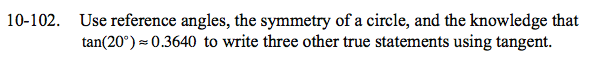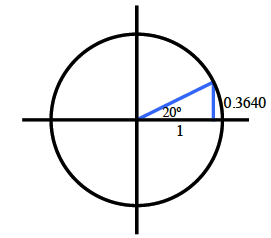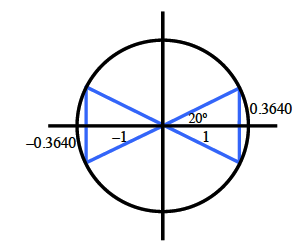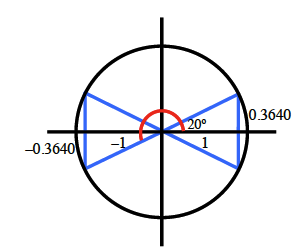Home > CCA2 > Chapter 10 > Lesson 10.2.1 > Problem10-102

10-102.

Use reference angles, the symmetry of a circle, and the knowledge that tan(20°) ≈ 0.3640 to write three other true statements using tangent. Homework Help ✎Draw the angle and corresponding right triangle in the first quadrant. Recall that tangent isopposite/adjacent, and label the side lengths.

Redraw your triangle in each of the other quadrants. Label the side lengths with the correct (±) sign.

$\text{One possible statement: tan}(200^{\circ})=\frac{-.3640}{-1}=0.3640$